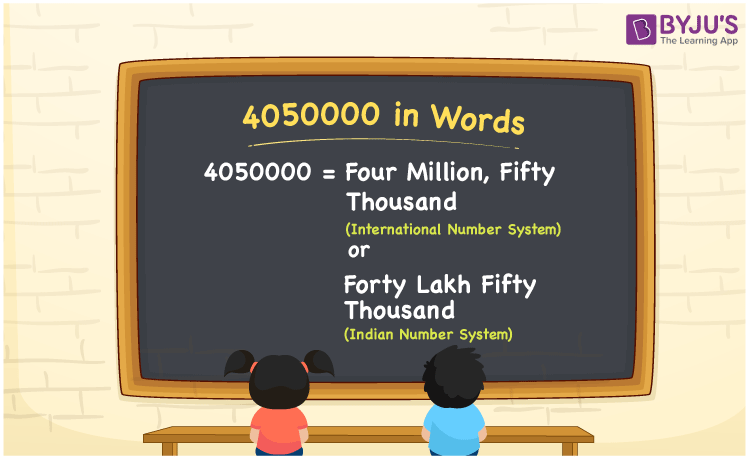# 4050000 in Words

We can write the number 4050000 in words as Forty lakh fifty thousand in the Indian number system and Four million fifty thousand in the International number system. In this article, you will learn how to write the cardinal number 4050000 in words using both International and Indian number systems using simple techniques.

 4050000 in words Four million fifty thousand  (or) Forty lakh fifty thousand Four million fifty thousand  (or) Forty lakh fifty thousand in Numbers 4050000

## 4050000 in English Words

We generally express numbers in words using the English alphabet. Thus, we spell 4050000 in English as “Forty lakh fifty thousand or Four million fifty thousand”.## How to Write 4050000 in Words?

4050000 in words – International number system

The below table depicts the place value chart for the numeral 4050000 for the International number system.

 Millions Hundred thousands Ten thousands Thousands Hundreds Tens Ones 4 0 5 0 0 0 0

Here, ones = 0, tens = 0, hundreds = 0, thousands = 0, ten thousands = 5, hundred thousands = 0, millions = 4

So, 4 × million + 0 × hundred thousand + 5 × ten thousand + 0 × thousand + 0 × hundred + 0 × tens + 0 × ones

= 4 million + 50 thousand

= Four million + Fifty thousand

Therefore, 4050000 in words = Four million fifty thousand.

4050000 in words – Indian number system

The below table illustrates the place value chart for 4050000 in the Indian number system.

 Ten lakhs Lakhs Ten thousands Thousands Hundreds Tens Ones 4 0 5 0 0 0 0

Here, ones = 0, tens = 0, hundreds = 0, thousands = 0, ten thousands = 5, lakhs = 0, ten lakhs = 4

The above digits can be written in the expanded form as:

= 4 × ten lakh + 0 × lakh + 5 × ten thousand + 0 × thousand + 0 × hundred + 0 × tens + 0 × ones

= 4 × 1000000 + 5 × 10000

= Forty lakh + Fifty thousand

=Forty lakh fifty thousand

Therefore, 4050000 in words = Forty lakh fifty thousand.

4050000 is a natural number that precedes 4050001 and succeeds 4049999.

4050000 in words – Forty lakh fifty thousand (or) Four million fifty thousand

Is 4050000 an odd number? – No

Is 4050000 an even number? – Yes

Is 4050000 a perfect square number? – No

Is 4050000 a perfect cube number? – No

Is 4050000 a prime number? – No

Is 4050000 a composite number? – Yes

## Frequently Asked Questions on 4050000 in Words

Q1

### How do you write 4050000 in words?

We can write the 4050000 in words as Four million fifty thousand or Forty lakh fifty thousand.
Q2

### How to write an amount of Rs. 4050000 in words on a cheque?

On a cheque, we generally write an amount of Rs. 4050000 in words as “Four million fifty thousand rupees only or Forty lakh fifty thousand rupees only”.
Q3

### Write 4050000 in standard form.

The standard form of 4050000 is 4.05 × 10^6.